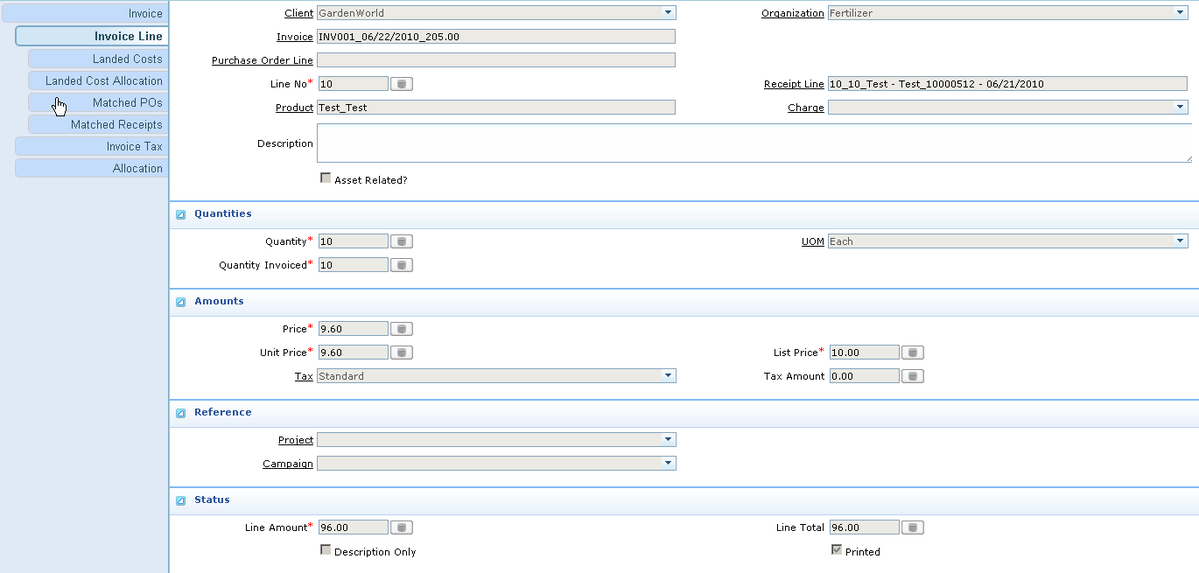# Attribute Level Pricing

August 25, 2023
• Community
• Wiki
• Developer's Guide

Enable the system to calculate purchase price based on product's attributes, using rule defined in the system. It will be easier to modify/calculate new price.

Customized by FMISopen in new window

## # Case Study

We have a product: Test, which has 3 attributes: TN1 (Number), TN2 (Number) and TL1 (List). Price for the product "Test" is as follows: Base Price: 10\$

For Attribute TL1

If TL1="Good", bonus of 0.5\$, otherwise, deduction of 0.3

For Attribute TN1

0`<TN1<1: Deduction of 0.1`

1<=TN1<2: No bonus/deduction

2<=TN1<3: Bonus of 0.1

3<=TN1: bonus of 0.2

For Attribute TN2

TN2 <=3: No bonus/deduction

3`<TN2: Step bonus of 0.2 for every increase of 1.`

We calculate the price at the end of month for all receipts within that month.

## # Customization

### # Create Rule: TestPricing

double tn1=getAttributeNumber("TN1");

double tn2=getAttributeNumber("TN2");

String tl1=getAttributeString("TL1");

double result1=0;

double result2=0;

double result3=0;

if `(0<tn1 && tn1<1) result1=-0.1;`

if (2<=tn1 && tn1<3) result1=0.1;

if (3<=tn1) result1=0.2;

if (tn2<=3) result2=0;

if `(3<tn2) result2 = Math.ceil(tn2-3)*0.2;`

if (tl1.equals("Good")) result3=0.5; else result3=-0.3;

result=result1+result2+result3;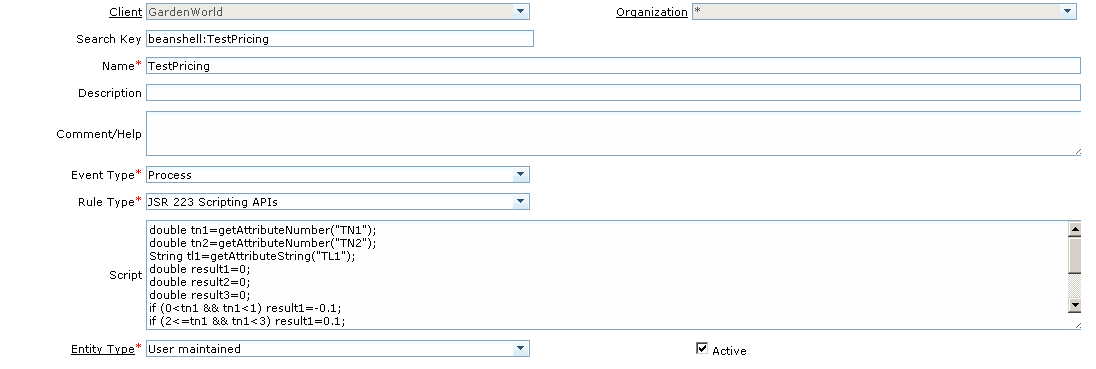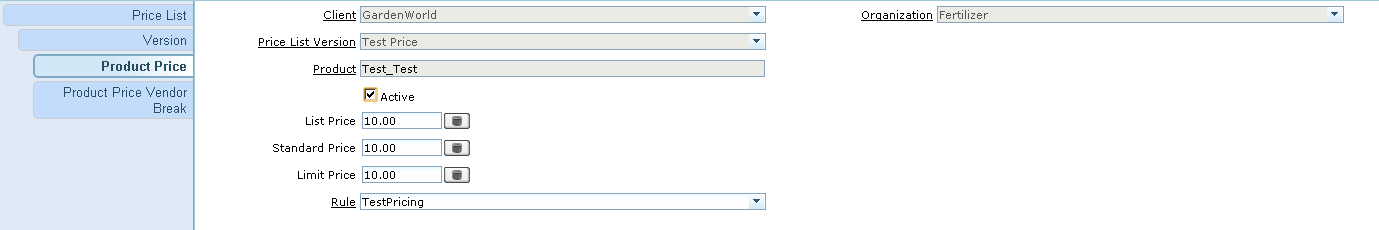### # Material Receipt

Since we calculate purchase price at the end of month, we have create a "Material Receipt Batch" windows, which covers all tabs from the original "Material Receipt" windows, with a process to create Vendor Invoice for all receipts within the batch windows.

#### # Material Receipt Batch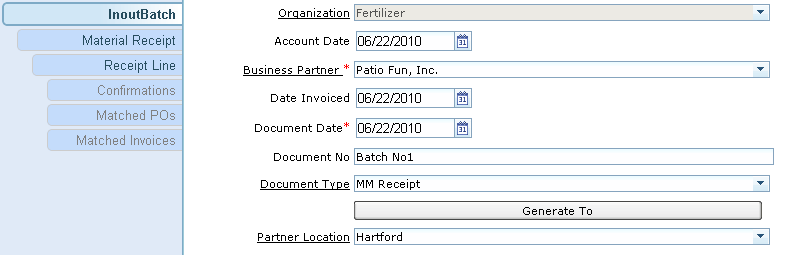#### # Material Receipt 1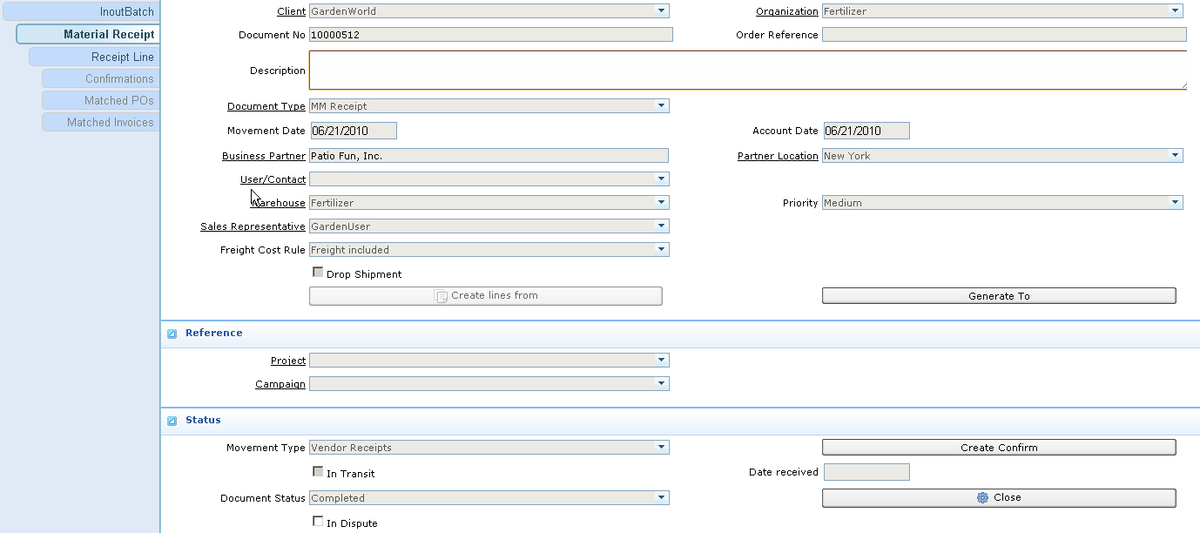#### # We enter the 1st attribute set for our Test Product: AL1="Pass", AN1=0.2, AN2=2.9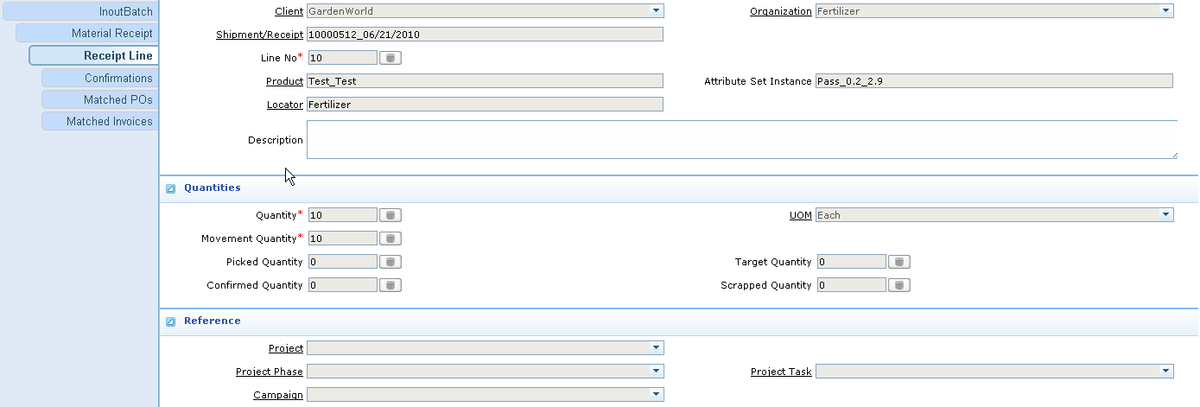#### # Material Receipt 2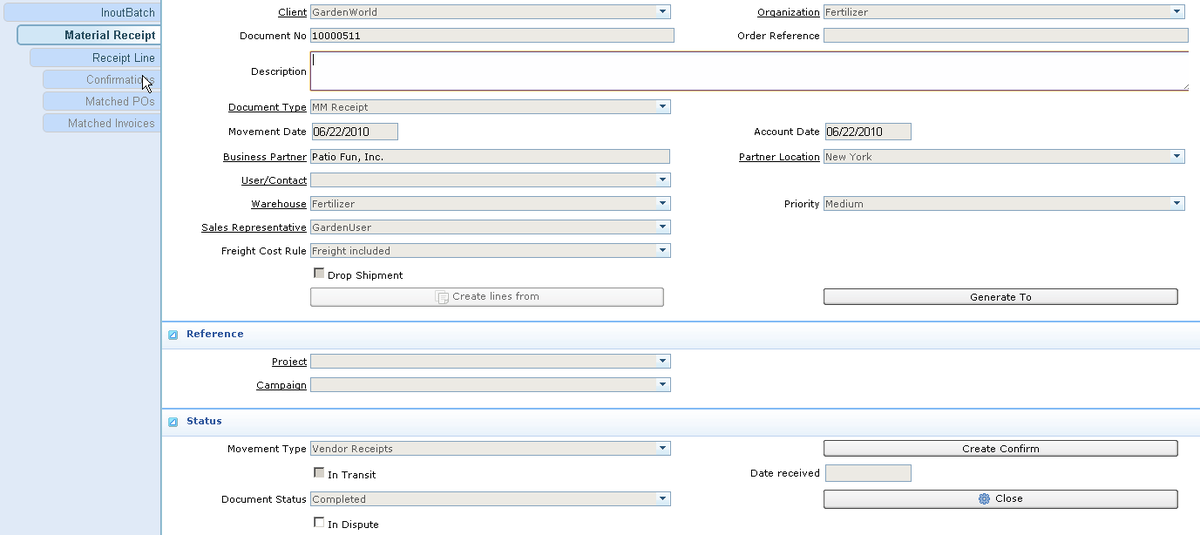#### # We enter the 2nd attribute set for our Test Product: AL2="Good", AN1=3, AN2=3.1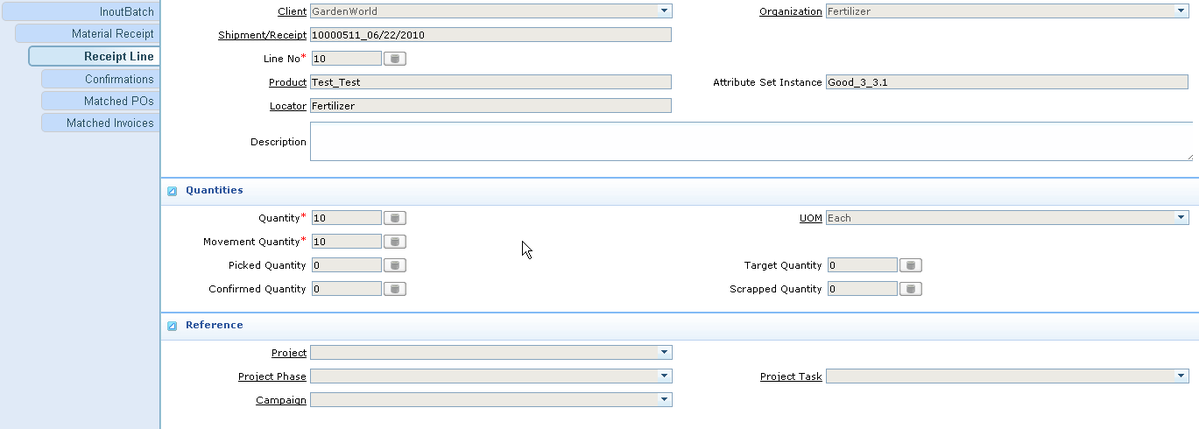We can complete all Material Receipt as normal

### # Create Vendor Invoice

We then run the "Generate To" button in the InoutBatch to create Vendor Invoice.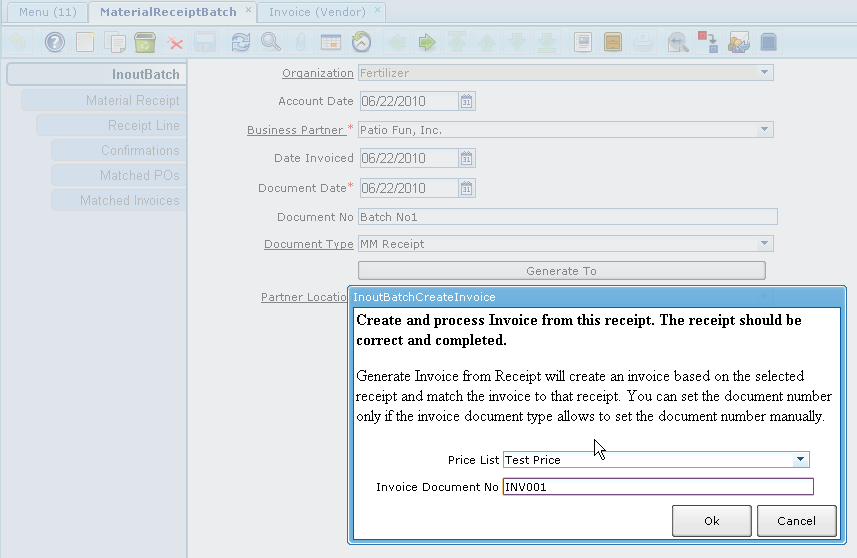### # Check Price

Vendor Invoice Line 1 (2nd Receipt), Price = 10.9 = 10 + 0.5 (TL1) + 0.2 (TN2) + 0.2 (TN2)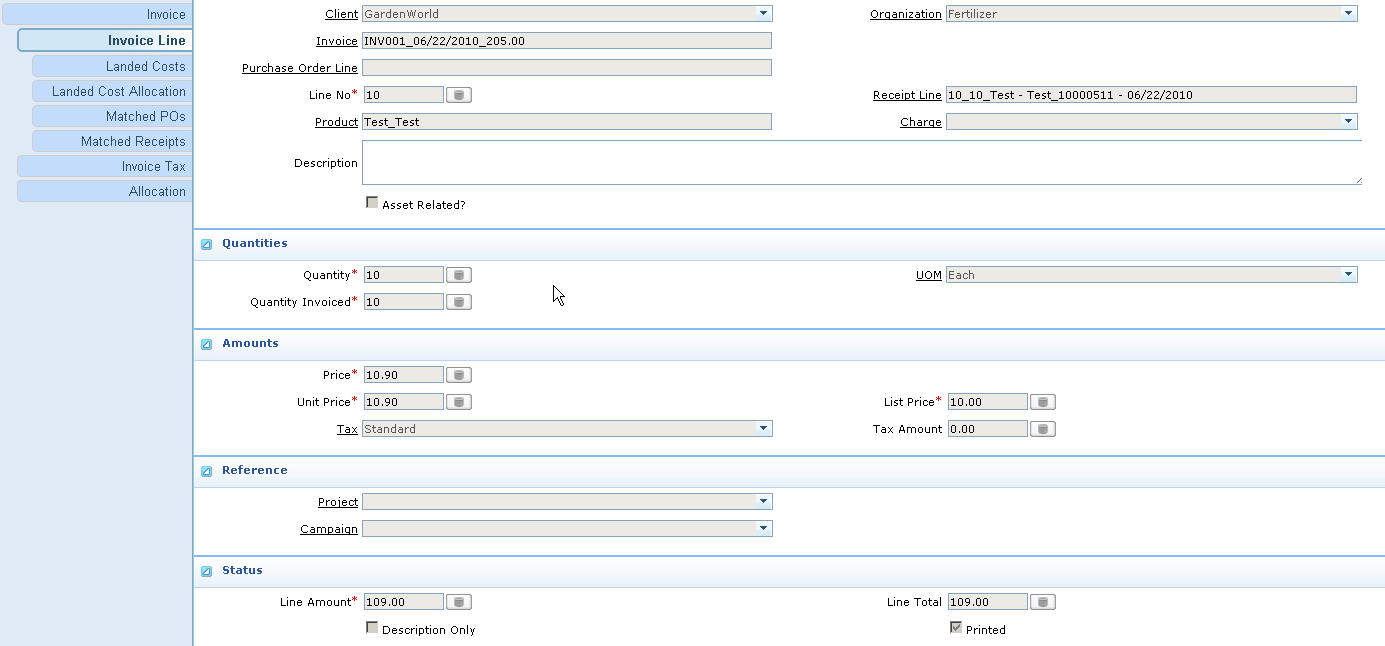Vendor Invoice Line 2 (1st Receipt), Price = 9.8 = 10 - 0.3 (TL1) - 0.1 (TN1) - 0 (TN2)